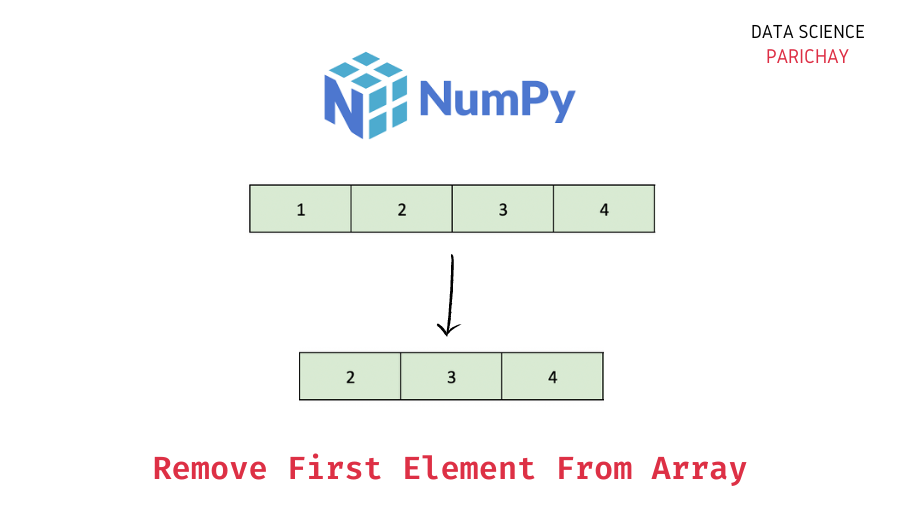# Remove First Element From Numpy Array

In this tutorial, we will look at how to remove the first element from a Numpy array with the help of some examples.

## How do I remove the first element of a Numpy Array?

You can use the numpy `delete()` function to remove the first element of a numpy array. Pass `0` as the index of the element to be removed. The following is the syntax –

```# remove first element from numpy array ar, assuming numpy imported as np
np.delete(ar, 0)```

The `np.delete()` function is used to remove an element using its index. Since we want to remove the first element, we pass `0`. It returns a copy of the original array with the specific element deleted.

Alternatively, you can also slice the original array starting from the index `1` to the end of the array to get a new numpy array with the first element removed from the original array.

Note that numpy arrays are immutable. That is, they cannot be modified after creation.

## Steps to remove the first element in Numpy

Let’s now look at a step-by-step example of using the methods mentioned above –

### Step 1 – Create the numpy array

First, we will create a numpy array that we will be using throughout this tutorial.

```import numpy as np

# create numpy array
ar = np.array([10, 20, 30, 40, 50])
# print the array
print(ar)```

Output:

📚 Data Science Programs By Skill Level

Introductory

Intermediate ⭐⭐⭐

🔎 Find Data Science Programs 👨‍💻 111,889 already enrolled

Disclaimer: Data Science Parichay is reader supported. When you purchase a course through a link on this site, we may earn a small commission at no additional cost to you. Earned commissions help support this website and its team of writers.

`[10 20 30 40 50]`

Here we use the `np.array()` function to create a numpy array from a list of numbers. We now have a numpy array containing the numbers 10, 20, 30, 40, and 50.

### Step 2 – Remove the first element from the array

First, let’s use the `np.delete()` function to remove the first element of the numpy array `ar` created above.

```# remove first element
ar_new = np.delete(ar, 0)
# print the returned array
print(ar_new)
# print the original array
print(ar)```

Output:

```[20 30 40 50]
[10 20 30 40 50]```

The resulting array does not have the first element from the original array. You can also see that the original array is not modified.

Let’s now use the slicing method to remove the first element.

```# remove first element
ar_new = ar[1:]
# print the returned array
print(ar_new)
# print the original array
print(ar)```

Output:

```[20 30 40 50]
[10 20 30 40 50]```

Here, we slice the original array from the index `1` to the end of the array. We get the same result as above. A copy of the original array with the first element removed.

For more on slicing numpy arrays, refer to numpy’s guide on indexing and slicing.

You might also be interested in –

••